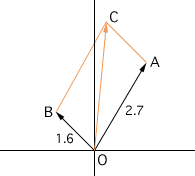Name: lena Subject: trigonometry Who is asking: Student Level: Secondary Question: how do you add vectors together? If you are given the length and angles of both vectors and are asked to add/subtract them, how do you do it? I know you are supposed to do the head to tail method, but whenever i try it i get the wrong answer. I need help setting it up. example: A is 2.7cm, and 60 degrees, B is 1.6cm and 135 degrees, find the magnitude and amplitude Thanks a lot! Hi Lena, Here is my diagram.From the diagram and the values given, |OA| = 2.7 cm, |OB| = |AC| = 1.6 cm and the angle OAC has measure 105o. You can find the length of OC using the law of cosines. |OC|2 = |OA|2 + |AC|2 - 2 |OA||AC| cos(angle OAC) = 2.72 + 1.62 - 2 x 2.7 x 1.6 cos(105o) Since cos(105o)is negative, |OC|2 is larger than 2.72 + 1.62. I got |OC| = 3.45 cm. To finish the problem you need to find the measure of the angle COA. This you can find using the law of sines,  sin(COA)/|AC| = sin(OAC)/|OC| I hope this helps, Penny Go to Math Central